# Subtracting Two Vectors

Subtracting Two Vectors

Suppose that $$\vec a$$ and $$\vec b$$ are two vectors. How can we interpret the subtraction of these vectors? That is, what meaning do we attach to $$\vec a - \vec b$$?

To start with, we note that $$\vec a - \vec b$$will be a vector which when added to $$\vec b$$ should give back $$\vec a$$:

$\left( {\vec a - \vec b} \right) + \vec b = \vec a$

But how do we determine the vector $$\vec a - \vec b$$, given the vectors  and $$\vec b$$? The following figure shows vectors $$\vec a$$ and $$\vec b$$ (we have drawn them to be co-initial):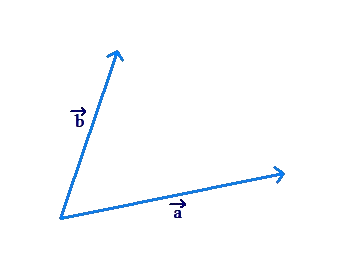We need a way to determine the vector $$\vec a - \vec b$$.

(i) Using the parallelogram law of vector addition, we can determine the vector as follows. We interpret $$\vec a - \vec b$$ as $$\vec a + \left( { - \vec b} \right)$$, that is, the vector sum of $$\vec a$$ and $$- \vec b$$. Now, we reverse vector $$\vec b$$, and then add $$\vec a$$ and $$- \vec b$$ using the parallelogram law: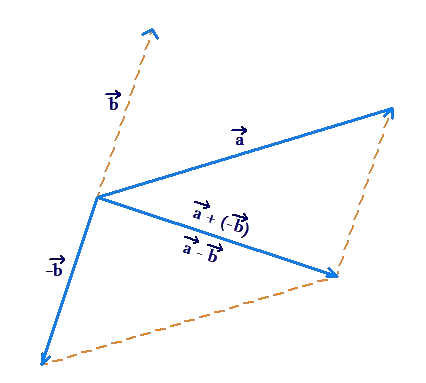(ii) We can also use the triangle law of vector addition. Denote the vector drawn from the end-point of $$\vec b$$ to the end-point of $$\vec a$$ by $$\vec c$$: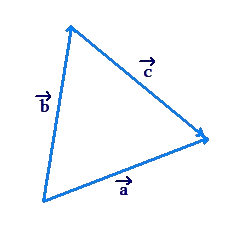Note that $$\vec b + \vec c = \vec a\,$$. Thus, $$\vec c = \vec a\, - \vec b$$. In other words, the vector $$\vec a - \vec b$$ is the vector drawn from the tip of $$\vec b$$ to the tip of $$\vec a$$ (if $$\vec a$$ and $$\vec b$$ are co-initial).

Note that both ways described above give us the same vector for $$\vec a - \vec b$$. This becomes clearer from the figure below: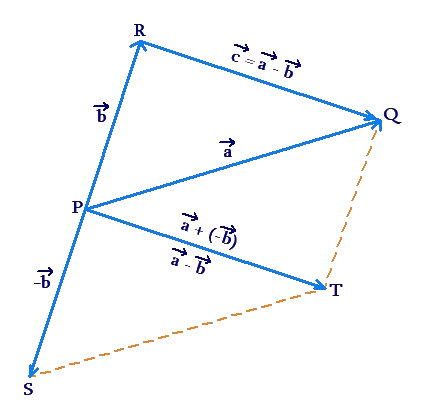The vector $$\overrightarrow {PT}$$ is obtained by adding $$\vec a$$ and $$- \vec b$$ using the parallelogram law. The vector $$\overrightarrow {RQ}$$ is obtained by drawing the vector from the tip of $$\vec b$$ to the tip of $$\vec a$$. Clearly, both vectors are the same (they are translated versions of each other).

Example 1: Vector $$\vec a$$ has a magnitude of 2 units and points towards the west. Vector $$\vec b$$ has a magnitude of 2 units and makes an angle of 1200 with the east direction: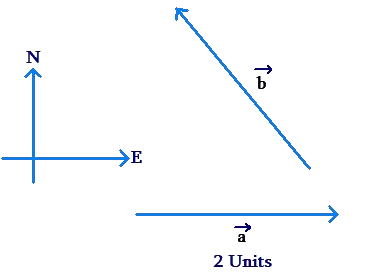Find $$\vec a\, - \vec b$$.

Solution: Make $$\vec a$$ and $$\vec b$$ co-initial, and draw the vector from the tip of $$\vec b$$ to the tip of $$\vec a$$: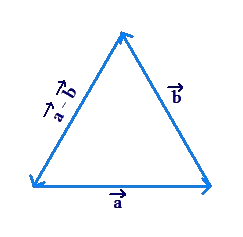Clearly, the triangle formed by these three vectors is equilateral. Thus, $$\vec a\, - \vec b$$ is a vector of magnitude 2 units, and makes an angle of 1200 with the east direction, measured in a clockwise manner: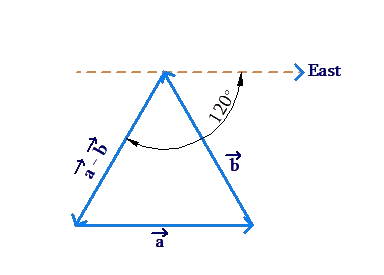Example 2: A unit vector is a vector with unit magnitude. A unit vector is generally denoted by a cap on top of a letter. For example, whenever you encounter symbols like $$\widehat a$$, $$\widehat b$$, $$\widehat c$$ etc., you should interpret these as unit vectors. $$\widehat a$$ and $$\widehat b$$ are two unit vectors inclined at an angle of $$\theta$$ to each other: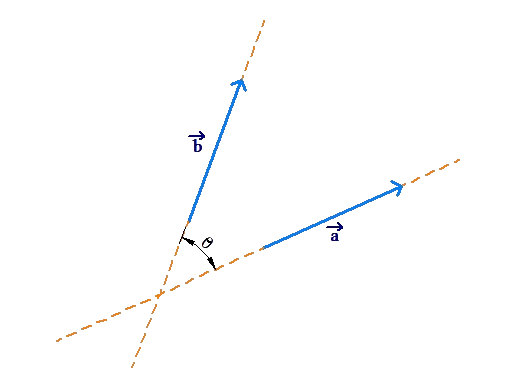Find $$\left| {\widehat a - \widehat b} \right|$$.

Solution: We need to find the magnitude of $$\widehat a - \widehat b$$. Let us make $$\widehat a$$ and $$\widehat b$$ co-initial, and draw the vector $$\vec c$$ from the tip of $$\widehat b$$ to the tip of $$\widehat a$$, as shown below: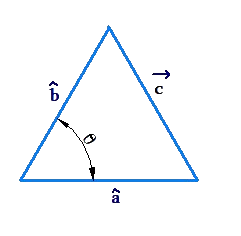To find the magnitude of $$\vec c$$, we use the cosine law:

\begin{align}&\left| {\overrightarrow c } \right| = \sqrt {{{\left| {\widehat a} \right|}^2} + {{\left| {\widehat b} \right|}^2} - 2\left| {\widehat a} \right|\left| {\widehat b} \right|\cos \theta } \\\,\,\, &\;\;\;\;\;= \sqrt {1 + 1 - 2\left( 1 \right)\left( 1 \right)\cos \theta } \\\,\,\, &\;\;\;\;\;= \sqrt {2 - 2\cos \theta } = \sqrt {2\left( {1 - \cos \theta } \right)} \\\,\,\, &\;\;\;\;\;= \sqrt {4{{\sin }^2}\frac{\theta }{2}} = 2\sin \frac{\theta }{2}\end{align}

Example 3: What can you say about non-zero vectors $$\vec a$$ and $$\vec b$$, if $$\left| {\vec a\, + \vec b} \right| = \left| {\vec a\, - \vec b} \right|$$?

Solution: The relation $$\left| {\vec a\, + \vec b} \right| = \left| {\vec a\, - \vec b} \right|$$ says that the magnitude of the sum of vectors $$\vec a$$ and $$\vec b$$ is equal to the magnitude of their difference. Consider the following figure:Note that in this particular figure, vectors $$\vec a\, + \vec b$$ and $$\vec a\, - \vec b$$ have unequal lengths. Is there any case possible when the two have equal lengths? A little thinking will show that this is possible only when $$\vec a$$ and $$\vec b$$ are perpendicular. In such a scenario, $$\vec b$$ and $$- \vec b$$ have a symmetry about $$\vec a$$: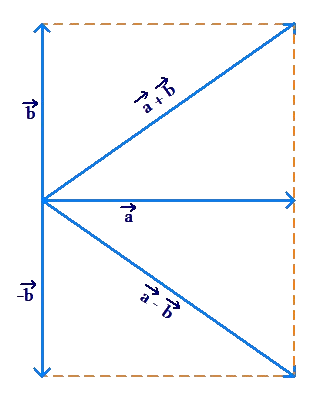Clearly, $$\vec a\, + \vec b$$ and $$\vec a\, - \vec b$$ have equal lengths in this case. Thus, for two non-zero vectors $$\vec a$$ and $$\vec b$$, $$\left| {\vec a\, + \vec b} \right| = \left| {\vec a\, - \vec b} \right|$$ only if $$\vec a$$ and $$\vec b$$ are perpendicular.

Vectors
grade 10 | Questions Set 1
Vectors
Vectors
grade 10 | Questions Set 2
Vectors
More Important Topics
Numbers
Algebra
Geometry
Measurement
Money
Data
Trigonometry
Calculus
More Important Topics
Numbers
Algebra
Geometry
Measurement
Money
Data
Trigonometry
Calculus